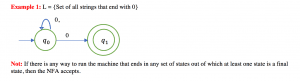Example of Conversion of Non-Deterministic Finite Automata (NFA) to Deterministic Finite Automata (DFA) – 2

Example :  L = {Set of all string over (0,1) that ends with 1}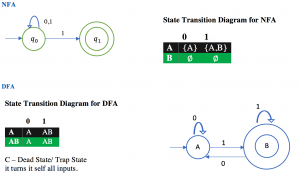Example of Conversion of Non-Deterministic Finite Automata (NFA) to Deterministic Finite Automata (DFA) – 1

Example:  L= {Set of all strings over (0,1) that start with 0}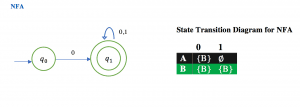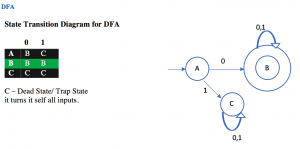Example of Non-Deterministic Finite Automata (NFA) – 8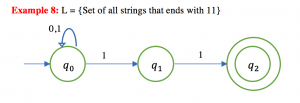Example of Non-Deterministic Finite Automata (NFA) – 7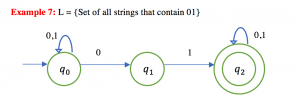Example of Non-Deterministic Finite Automata (NFA) – 6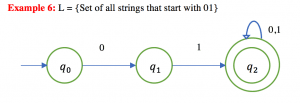Example of Non-Deterministic Finite Automata (NFA) – 5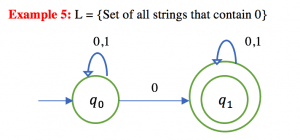Example of Non-Deterministic Finite Automata (NFA) – 4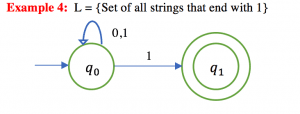Example of Non-Deterministic Finite Automata (NFA) – 3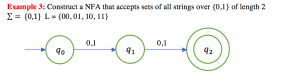Example of Non-Deterministic Finite Automata (NFA) – 2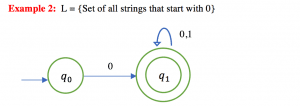Example of Non-Deterministic Finite Automata (NFA) – 1